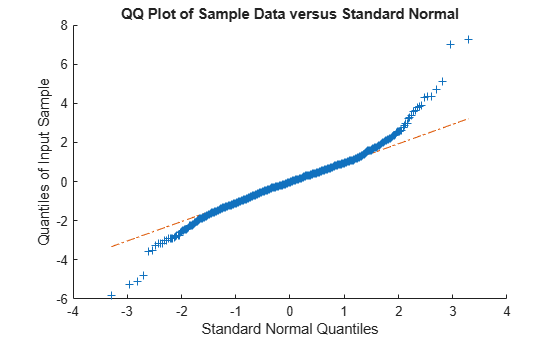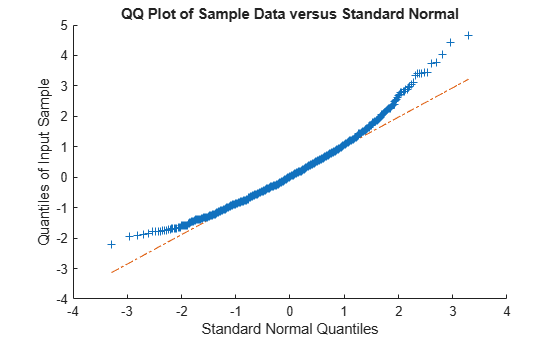# johnsrnd

Johnson system random numbers

## Syntax

```r = johnsrnd(quantiles,m,n) r = johnsrnd(quantiles) [r,type] = johnsrnd(...) [r,type,coefs] = johnsrnd(...) ```

## Description

`r = johnsrnd(quantiles,m,n)` returns an `m`-by-`n` matrix of random numbers drawn from the distribution in the Johnson system that satisfies the quantile specification given by `quantiles`. `quantiles` is a four-element vector of quantiles for the desired distribution that correspond to the standard normal quantiles [–1.5 –0.5 0.5 1.5]. In other words, you specify a distribution from which to draw random values by designating quantiles that correspond to the cumulative probabilities [0.067 0.309 0.691 0.933]. `quantiles` may also be a `2`-by-`4` matrix whose first row contains four standard normal quantiles, and whose second row contains the corresponding quantiles of the desired distribution. The standard normal quantiles must be spaced evenly.

### Note

Because `r` is a random sample, its sample quantiles typically differ somewhat from the specified distribution quantiles.

`r = johnsrnd(quantiles)` returns a scalar value.

`r = johnsrnd(quantiles,m,n,...)` or ```r = johnsrnd(quantiles,[m,n,...])``` returns an `m`-by-`n`-by-... array.

`[r,type] = johnsrnd(...)` returns the type of the specified distribution within the Johnson system. `type` is `'SN'`, `'SL'`, `'SB'`, or `'SU'`. Set `m` and `n` to zero to identify the distribution type without generating any random values.

The four distribution types in the Johnson system correspond to the following transformations of a normal random variate:

• `'SN'` — Identity transformation (normal distribution)

• `'SL'` — Exponential transformation (lognormal distribution)

• `'SB'` — Logistic transformation (bounded)

• `'SU'` — Hyperbolic sine transformation (unbounded)

`[r,type,coefs] = johnsrnd(...)` returns coefficients `coefs` of the transformation that defines the distribution. `coefs` is ```[gamma, eta, epsilon, lambda]```. If `z` is a standard normal random variable and `h` is one of the transformations defined above, `r = lambda*h((z-gamma)/eta)+epsilon` is a random variate from the distribution type corresponding to `h`.

## Examples

collapse all

This example shows several different approaches to using the Johnson system of flexible distribution families to generate random numbers and fit a distribution to sample data.

Generate random values with longer tails than a standard normal.

```rng default; % For reproducibility r = johnsrnd([-1.7 -.5 .5 1.7],1000,1); figure; qqplot(r);```Generate random values skewed to the right.

```r = johnsrnd([-1.3 -.5 .5 1.7],1000,1); figure; qqplot(r);```Generate random values that match some sample data well in the right-hand tail.

```load carbig; qnorm = [.5 1 1.5 2]; q = quantile(Acceleration, normcdf(qnorm)); r = johnsrnd([qnorm;q],1000,1); [q;quantile(r,normcdf(qnorm))]```
```ans = 2×4 16.7000 18.2086 19.5376 21.7263 16.6986 18.2220 19.9078 22.0918 ```

Determine the distribution type and the coefficients.

`[r,type,coefs] = johnsrnd([qnorm;q],0)`
```r = [] ```
```type = 'SU' ```
```coefs = 1×4 1.0920 0.5829 18.4382 1.4494 ```